# Traditional and Modern Theory of Cost in Short Run and Long Run

Traditional theory distinguishes between the short run and the long run. The short run is the period during which some factors) is fixed; usually capital equipment and entrepreneurship are considered as fixed in the short run.

The long run is the period over which all factors become variable.

1. Short-Run Costs of the Traditional Theory:

In the traditional theory of the firm total costs are split into two groups total fixed costs and total variable costs:

TC = TFC + TVC

The fixed costs include:

(b) Depreciation (wear and tear) of machinery

(c) Expenses for building depreciation and repairs

(d) Expenses for land maintenance and depreciation (if any).

Another element that may be treated in the same way as fixed costs is the normal profit, which is a lump sum including a percentage return on fixed capital and allowance for risk.

The variable costs include:

(a) The raw materials

(b) The cost of direct labour

(c) The running expenses of fixed capital, such as fuel, ordinary repairs and routine maintenance.

The total fixed cost is graphically denoted by a straight line parallel to the output axis (figure 4.1). The total variable cost in the traditional theory of the firm has broadly an inverse-S shape (figure 4.2) which reflects the law of variable proportions. According to this law, at the initial stages of production with a given plant, as more of the variable factors) is employed, its productivity increases and the average variable cost falls.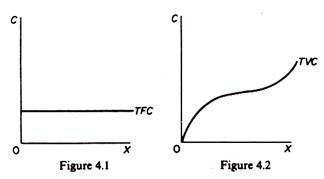This continues until the optimal combination of the fixed and variable factors is reached. Beyond this point as increased quantities of the variable factors(s) are combined with the fixed factors) the productivity of the variable factors) declines (and the A VC rises). By adding the TFC and TVC we obtain the TC of the firm (figure 4.3). From the total-cost curves we obtain average-cost curves.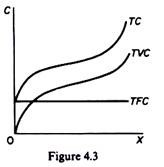The average fixed cost is found by dividing TFC by the level of output:

AFC = TFC / X

Graphically the AFC is a rectangular hyperbola, showing at all its points the same magnitude, that is, the level of TFC (figure 4.4).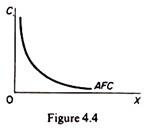The average variable cost is similarly obtained by dividing the TVC with the corresponding level of output:

AVC = TVC / X

Graphically the A VC at each level of output is derived from the slope of a line drawn from the origin to the point on the TVC curve corresponding to the particular level of output. For example, in figure 4.5 the AVC at X1 is the slope of the ray 0a, the A VC at X2 is the slope of the ray Ob, and so on. It is clear from figure 4.5 that the slope of a ray through the origin declines continuously until the ray becomes tangent to the TVC curve at c. To the right of this point the slope of rays through the origin starts increasing. Thus the SA VC curve falls initially as the productivity of the variable factors) increases, reaches a minimum when the plant is operated optimally (with the optimal combination of fixed and variable factors), and rises beyond that point (figure 4.6).The ATC is obtained by dividing the TC by the corresponding level of output:

ATC = TC / X = TFC + TVC / X = AFC + AVC

Graphically the ATC curve is derived in the same way as the SAVC. The ATC at any level of output is the slope of the straight line from the origin to the point on the TC curve corresponding to that particular level of output (figure 4.7). The shape of the A TC is similar to that of the AVC (both being U-shaped). Initially the ATC declines, it reaches a minimum at the level of optimal operation of the plant (XM) and subsequently rises again (figure 4.8).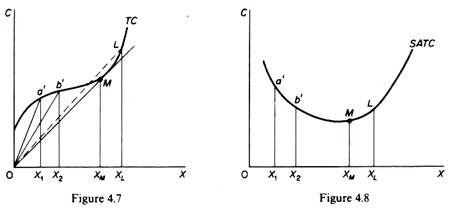The U shape of both the AVC and the ATC reflects the law of variable proportions or law of eventually decreasing returns to the variable factor(s) of production. The marginal cost is defined as the change in TC which results from a unit change in output. Mathematically the marginal cost is the first derivative of the TC function. Denoting total cost by C and output by X we have

MC = ∂C / ∂X

Graphically the MC is the slope of the TC curve (which of course is the same at any point as the slope of the TVC). The slope of a curve at any one of its points is the slope of the tangent at that point. With an inverse-S shape of the TC (and TVC) the MC curve will be U-shaped. In figure 4.9 we observe that the slope of the tangent to the total-cost curve declines gradually, until it becomes parallel to the X-axis (with its slope being equal to zero at this point), and then starts rising. Accordingly we picture the MC curve in figure 4.10 as U-shaped.In summary: the traditional theory of costs postulates that in the short run the cost curves (AVC, ATC and MC) is U-shaped, reflecting the law of variable proportions. In the short run with a fixed plant there is a phase of increasing productivity (falling unit costs) and a phase of decreasing productivity (increasing unit costs) of the variable factor(s).

Between these two phases of plant operation there is a single point at which unit costs are at a minimum. When this point on the SATC is reached the plant is utilized optimally, that is, with the optimal combination (proportions) of fixed and variable factors.

The relationship between ATC and AVC:

The AVC is a part of the ATC, given ATC = AFC + AVC. Both AVC and ATC are U-shaped, reflecting the law of variable proportions. However, the minimum point of the ATC occurs to the right of the minimum point of the AVC (figure 4.11). This is due to the fact that ATC includes AFC, and the latter falls continuously with increases in output.

After the AVC has reached its lowest point and starts rising, its rise is over a certain range offset by the fall in the AFC, so that the ATC continues to fall (over that range) despite the increase in AVC. However, the rise in AVC eventually becomes greater than the fall in the AFC so that the A TC starts increasing. The A VC approaches the A TC asymptotically as X increases.

In figure 4.11 the minimum AVC is reached at X1 while the ATC is at its minimum at X2. Between X1 and X2 the fall in AFC more than offsets the rise in AVC so that the ATC continues to fall. Beyond X2 the increase in AVC is not offset by the fall in AFC, so that ATC rises.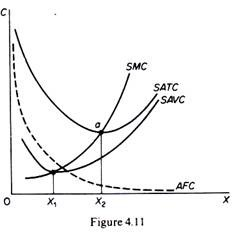The relationship between MC and ATC:

The MC cuts the ATC and the AVC at their lowest points. We will establish this relation only for the ATC and MC, but the relation between MC and AVC can be established on the same lines of reasoning.

We said that the MC is the change in the TC for producing an extra unit of output. Assume that we start from a level of n units of output. If we increase the output by one unit the MC is the change in total cost resulting from the production of the (n + l)th unit.

The AC at each level of output is found by dividing TC by X. Thus the AC at the level of Xis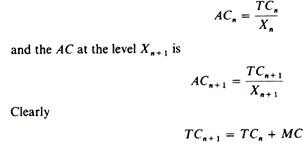Thus:

(a) If the MC of the (n + 1)th unit is less than ACn (the AC of the previous n units) the AC n+1 will be smaller than the ACn.

(b) If the MC of the (n + 1)th unit is higher than ACn (the AC of the previous n units) the ACn+1 will be higher than the ACn.

So long as the MC lies below the AC curve, it pulls the latter downwards; when the MC rises above the AC, it pulls the latter upwards. In figure 4.11 to the left of a the MC lies below the AC curve, and hence the latter falls downwards. To the right of a the MC curve lie above the AC curve, so that AC rises. It follows that at point a, where the inter­section of the MC and AC occurs, the AC has reached its minimum level.

1. Long-Run Costs of the Traditional Theory: The ‘Envelope’ Curve:

In the long run all factors are assumed to become variable. We said that the long-run cost curve is a planning curve, in the sense that it is a guide to the entrepreneur in his decision to plan the future expansion of his output. The long-run average-cost curve is derived from short-run cost curves. Each point on the LAC corresponds to a point on a short-run cost curve, which is tangent to the LAC at that point. Let us examine in detail how the LAC is derived from the SRC curves.

Assume, as a first approximation, that the available technology to the firm at a particular point of time includes three methods of production, each with a different plant size: a small plant, medium plant and large plant. The small plant operates with costs denoted by the curve SAC1, the medium-size plant operates with the costs on SAC2 and the large-size plant gives rise to the costs shown on SAC3 (figure 4.12). If the firm plans to produce output X3 it will choose the small plant. If it plans to produce X2 it will choose the medium plant. If it wishes to produce X1 it will choose the large- size plant.If the firm starts with the small plant and its demand gradually increases, it will produce at lower costs (up to level X’1). Beyond that point costs start increasing. If its demand reaches the level X”1 the firm can either continue to produce with the small plant or it can install the medium-size plant. The decision at this point depends not on costs but on the firm’s expectations about its future demand. If the firm expects that the demand will expand further than X”1 it will install the medium plant, because with this plant outputs larger than X’1 are produced with a lower cost.

Similar con­siderations hold for the decision of the firm when it reaches the level X”2. If it expects its demand to stay constant at this level, the firm will not install the large plant, given that it involves a larger investment which is profitable only if demand expands beyond X”2. For example, the level of output X3 is produced at a cost c3 with the large plant, while it costs c’2 if produced with the medium-size plant (c’2 > c3).

Now if we relax the assumption of the existence of only three plants and assume that the available technology includes many plant sizes, each suitable for a certain level of output, the points of intersection of consecutive plants (which are the crucial points for the decision of whether to switch to a larger plant) are more numerous. In the limit, if we assume that there is a very large number (infinite number) of plants, we obtain a continuous curve, which is the planning LAC curve of the firm.

Each point of this curve shows the minimum (optimal) cost for producing the corresponding level of output. The LAC curve is the locus of points denoting the least cost of producing the corresponding output. It is a planning curve because on the basis of this curve the firm decides what plant to set up in order to produce optimally (at minimum cost) the expected level of output.

The firm chooses the short-run plant which allows it to produce the anticipated (in the long run) output at the least possible cost. In the traditional theory of the firm the LAC curve is U-shaped and it is often called the ‘envelope curve’ because it ‘en­velopes’ the SRC curves (figure 4.13).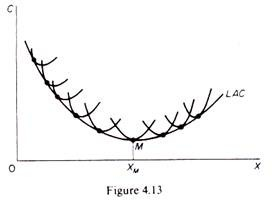Let us examine the U shape of the LAC. This shape reflects the laws of returns to scale. According to these laws the unit costs of production decrease as plant size increases, due to the economies of scale which the larger plant sizes make possible. The traditional theory of the firm assumes that economies of scale exist only up to a certain size of plant, which is known as the optimum plant size, because with this plant size all possible economies of scale are fully exploited.

If the plant increases further than this optimum size there are diseconomies of scale, arising from managerial inefficiencies. It is argued that management becomes highly complex, managers are overworked and the decision-making process becomes less efficient. The turning-up of the LAC curve is due to managerial diseconomies of scale, since the technical diseconomies can be avoided by duplicating the optimum technical plant size.

A serious implicit assumption of the traditional U-shaped cost curves is that each plant size is designed to produce optimally a single level of output (e.g. 1000 units of X). Any departure from that X, no matter how small (e.g. an increase by 1 unit of X) leads to increased costs. The plant is completely inflexible. There is no reserve capacity, not even to meet seasonal variations in demand.

As a consequence of this assumption the LAC curve ‘envelopes’ the SRAC. Each point of the LAC is a point of tangency with the corresponding SRAC curve. The point of tangency occurs to the falling part of the SRAC curves for points lying to the left of the minimum point of the LAC since the slope of the LAC is negative up to M (figure 4.13) the slope of the SRMC curves must also be negative, since at the point of their tangency the two curves have the same slope.

The point of tangency for outputs larger than XM occurs to the rising part of the SRAC curves since the LAC rises, the SAC must rise at the point of their tangency with the LAC. Only at the minimum point M of the LAC is the corresponding SAC also at a minimum. Thus at the falling part of the LAC the plants are not worked to full capacity; to the rising part of the LAC the plants are overworked; only at the minimum point M is the (short-run) plant optimally employed.

We stress once more the optimality implied by the LAC planning curve each point represents the least unit-cost for producing the corresponding level of output. Any point above the LAC is inefficient in that it shows a higher cost for producing the correspon­ding level of output. Any point below the LAC is economically desirable because it implies a lower unit-cost, but it is not attainable in the current state of technology and with the prevailing market prices of factors of production. (Recall that each cost curve is drawn under a ceteris paribus clause, which implies given state of technology and given factor prices.)

The long-run marginal cost is derived from the SRMC curves, but does not ‘en­velope’ them. The LRMC is formed from points of intersection of the SRMC curves with vertical lines (to the X-axis) drawn from the points of tangency of the corresponding SAC curves and the LRA cost curve (figure 4.14). The LMC must be equal to the SMC for the output at which the corresponding SAC is tangent to the LAC. For levels of X to the left of tangency a the SAC > LAC.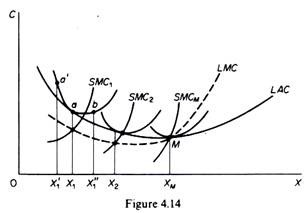At the point of tangency SAC = LAC. As we move from point a’ to a, we actually move from a position of inequality of SRAC and LRAC to a position of equality. Hence the change in total cost (i.e. the MC) must be smaller for the short-run curve than for the long-run curve. Thus LMC > SMC to the left of a. For an increase in output beyond X, (e.g. X’1) the SAC > LAC. That is, we move from the position a of equality of the two costs to the position b where SAC is greater than LAC. Hence the addition to total cost (= MC) must be larger for the short-run curve than for the long-run curve. Thus LMC < SMC to the right of a.

Since to the left of a, LMC > SMC, and to the right of a, LMC < SMC, it follows that at a, LMC – SMC. If we draw a vertical line from a to the X-axis the point at which it intersects the SMC (point A for SAC1) is a point of the LMC.

If we repeat this procedure for all points of tangency of SRAC and LAC curves to the left of the minimum point of the LAC, we obtain points of the section of the LMC which lies below the LAC. At the minimum point M the LMC intersects the LAC. To the right of M the LMC lies above the LAC curve. At point M we have

SACM = SMCM = LAC = LMC

There are various mathematical forms which give rise to U-shaped unit cost curves. The simplest total cost function which would incorporate the law of variable pro­portions is the cubic polynomial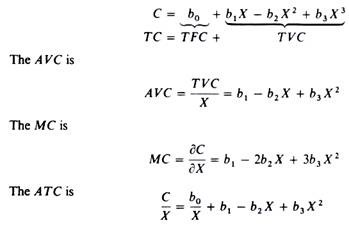The TC curve is roughly S-shaped , while the ATC, the AVC and the MC are all U-shaped; the MC curve intersects the other two curves at their minimum points (figure 4.11).

## The Modern Theory of Costs

The modem theory of costs differs from the traditional theory of costs with regard to the shapes of the cost curves. In the traditional theory, the cost curves are U-shaped. But in the modem theory which is based on empirical evidences, the short-run SAVC curve and the SMC curve coincide with each other and are a horizontal straight line over a wide range of output. So far as the LAC and LMC curves are concerned, they are L-shaped rather than U-shaped. We discuss below the nature of short- run and long-run cost curves according to the modem theory.

(1) Short-Run Cost Curves:

As in the traditional theory, the short-run cost curves in the modem theory of costs are the AFC, SAVC, SAC and SMC curves. As usual, they are derived from the total costs which are divided into total fixed costs and total variable costs.

But in the modem theory, the SAVC and SMC curves have a saucer-type shape or bowl-shape rather than a U-shape. As the AFC curve is a rectangular hyperbola, the SAC curve has a U-shape even in the modem version. Economists have investigated on the basis of empirical studies this behaviour pattern of the short-run cost curves.

According to them, a modern firm chooses such a plant which it can operate eas­ily with the available variable direct factors. Such a plant possesses some reserve capacity and much flexibility. The firm installs this type of plant in order to produce the maximum rate of output over a wide range to meet any increase in demand for its product.

The saucer-shaped SAVC and SMC curves are shown in Figure 7. To begin with, both the curves first fall upto point A and the SMC curvelies below the SAVC curve. “The falling part of the SAVC shows the reduction in costs due to the better utilisation of the fixed factor and the consequent increase in skills and productiv­ity of the variable factor (labour).With better skills, the wastes in raw materials are also being reduced and a better utilisation of the whole plant is reached.” So far as the flat stretch of the saucer-shaped SAVC curve over Q:1Q2 range of output is concerned, the empirical evidence reveals that the operation of a plant within this wide range exhibits constant returns to scale.

The reason for the saucer-shaped SAVC curve is that the fixed factor is divisible. The SAV costs are constant over a large range, up to the point at which all of the fixed factor is used. Moreover, the firm’s SAV costs tend to be constant over a wide range of output because there is no need to depart from the optimal combination of labour and capital in those plants that are kept in operation.

Thus there is a large range of output over which the SAVC curve will be flat. Over that range, SMC and SAVC are equal and are constant per unit of output. The firm will, therefore, continue to produce within Q1Q2 reserve capacity of the plant, as shown in Figure 7.

After point B, both the SAVC and SMC curves start rising. When the firm departs from its normal or the load factor of the plant in order to obtain higher rates of output beyond Q2, it leads to higher SAVC and SMC. The increase in costs may be due to the over­time operations of the old and less efficient plant leading to frequent breakdowns, wastage of raw materials, reduction in labour productivity and increase in labour cost due to overtime operations. In the rising portion of the SAVC curve beyond point B, the SMC curve lies above it.

The short-run average total cost curve (SATC or SAC) is obtained by adding vertically the average fixed cost curve (AFC) and the SAVC curve at each level of output. The SAC curve, as shown in Figure 8, continues to fall up to the OQ level of output at which the reserve capacity of the plant is fully exhausted.Beyond that output level, the SAC curve rises as output increases. The smooth and continuous fall in the SAC curve upto the OQ level of output is due to the fact that the AFC curve is a rectangular hyperbola and the SAVC curve first falls and then becomes horizontal within the range of reserve capacity. Beyond the OQ output level, it starts rising steeply. But the minimum point M of the SAC curve where the SMC curve intersects it, is to the right of point E of the SAVC curve. This is because the SAVC curve starts rising steeply from point E while the AFC curve is falling at a very low rate.

(2) Long-Run Cost Curves:

Empirical evidence about the long-run average cost curve reveals that the LAC curve is L-shaped rather than U-shaped. In the beginning, the LAC curve rapidly falls but after a point “the curve remains flat, or may slope gently downwards, at its right-hand end.” Economists have assigned the following reasons for the L-shape of the LAC curve.

1. Production and Managerial Costs:

In the long run, all costs being variable, production costs and managerial costs of a firm are taken into account when considering the effect of expansion of output on average costs. As output increases, production costs fall continuously while managerial costs may rise at very large scales of output. But the fall in production costs outweighs the increase in managerial costs so that the LAC curve falls with increases in output. We analyse the behaviour of production and managerial costs in explaining the L-shape of the LAC curve.

Production Costs:

As a firm increases its scale of production, its production costs fall steeply in the beginning and then gradually. The is due to the technical economies of large scale production enjoyed by the firm. Initially, these economies are substantial. But after a certain level of output when all or most of these economies have been achieved, the firm reaches the minimum optimal scale or mini­ mum efficient scale (MES).

Given the technology of the industry, the firm can continue to enjoy some technical economies at outputs larger than the MES for the following reasons:

(a) from further decentralisation and improvement in skills and productivity of labour; (b) from lower repair costs after the firm reaches a certain size; and

(c) by itself producing some of the materials and equipment cheaply which the firm ne

Managerial Costs:

In modern firms, for each plant there is a corresponding managerial set-up for its smooth operation. There are various levels of management, each having a separate management technique applicable to a certain range of output. Thus, given a managerial set-up for a plant, its mana­gerial costs first fall with the expansion of output and it is only at a very large scale output, they rise very slowly.

To sum up, production costs fall smoothly and managerial costs rise slowly at very large scales of output. But the fall in production costs more than offsets the rise in managerial costs so that the LAC curve falls smoothly or becomes flat at very large scales of output, thereby giving rise to the L-shape of the LAC curve.

In order to draw such an LAC curve, we take three short-run average cost curves SAC1 SA С2, and SAC3representing three plants with the same technol­ogy in Figure 9. Each SAC curve includes production costs, managerial costs, other fixed costs and a mar­gin for normal profits. Each scale of plant (SAC) is subject to a typical load factor capacity so that points A, В and С represent the minimal optimal scale of out­put of each plant.By joining all such points as A, В and С of a large number of SACs, we trace out a smooth and continuous LAC curve, as shown in Figure 9. This curve does not turn up at very large scales of output. It does not envelope the SAC curves but intersects them at the optimal level of output of each plant.

1. Technical Progress:

Another reason for the existence of the L-shaped LAC curve in the modern theory of costs is technical progress. The traditional theory of costs assumes no technical progress while explaining the U-shaped LAC curve. The empirical results on long-run costs conform the widespread existence of economies of scale due to technical progress in firms.

The period between which technical progress has taken place, the long-run aver­age costs show a falling trend. The evidence of diseconomies is much less certain. So an upturn of the LAC at the top end of the size scale has not been observed. The L-shape of the LAC curve due to tech­nical progress is explained in Figure 10.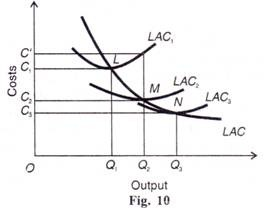Suppose the firm is producing OQ1 output on LAC1curve at a per unit cost of ОС1 If there is an increase in demand for the firm’s product to OQ2,with no change in technology, the firm will produce OQ2 output along the LAC1 curve at a per unit cost of ОС2. If, however, there is technical progress in the firm, it will install a new plant having LAC2 as the long-run average cost curve. On this plant, it produces OQ2 output at a lower cost OC2 per unit.

Similarly, if the firm decides to increase its output to OQ3 to meet further rise in demand technical progress may have advanced to such a level that it installs the plant with the LAC3 curve. Now it produces OQ3output at a still lower cost OC3 per unit. If the minimum points, L, M and N of these U- shaped long-run average cost curves LAC1, LAC2 and LAC3are joined by a line, it forms an L-shaped gently sloping downward curve LAC.

1. Learning:

Another reason for the L-shaped long- run average cost curve is the learning process. Learning is the product of experience. If experience, in this context, can be measured by the amount of a commodity produced, then higher the production is, the lower is per unit cost.

The consequences of learning are similar to increasing re­turns. First, the knowledge gained from working on a large scale cannot be forgotten. Second, learning increases the rate of productivity. Third, experience is measured by the aggregate output produced since the firm first started to produce the product.

Learning-by-doing has been observed when firms start producing new products. After they have produced the first unit, they are able to reduce the time required for production and thus reduce their per unit costs. For example, if a firm manufactures airframes, the fall observed in long-run average costs is a function of experience in producing one particular kind of airframe, not airframes in general.

One can, therefore, draw a “learning curve” which relates cost per airframe to the aggregate number of airframes manufactured so far, since the firm started manufacturing them. Figure 11 shows a learning curve LAC which relates the cost of producing a given output to the total output over the entire time period.

Growing experience with making the product leads to falling costs as more and more of it is produced. When the firm has exploited all learning possibilities, costs reach a minimum level, M in the figure. Thus, the LAC curve is L-shaped due to learning by doing.Relation between LAC and LMC Curves:

In the modern theory of costs, if the LAC curve falls smoothly and continuously even at very large scales of output, the LMC curve will lie below the LAC curve throughout its length, as shown in Figure 12.If the LAC curve is downward sloping up to the point of a minimum optimal scale of plant or a mini­mum efficient scale (MES) of plant beyond which no further scale economies exist, the LAC curve becomes horizontal. In this case, the LMC curve lies below the LAC curve until the MES point M is reached, and beyond this point the LMC curve coincides with the LA С curve, as shown in Figure 13.Conclusion:

The majority of empirical cost studies suggest that the U-shaped cost curves postulated by the traditional theory are not observed in the real world. Two major results emerge predominantly from most studies. First, the SAVC and SMC curves are constant over a wide-range of output.

Second, the LAC curve falls sharply over low levels of output, and subse­quently remains practically constant as the scale of output increases. This means that the LAC curve is L-shaped rather than U-shaped. Only in very few cases diseconomies of scale were observed, and these at very high levels of output.

Economies of Scale and the LAC Curve:

The shape of the LAC curve depends fundamentally upon the internal economies and diseconomies of scale, while the shift in the LAC curve depends upon external economies and diseconomies of scale. The LAC curve first declines slowly and then rises gradually after a minimum point is reached.

Initially, the LAC curve slopes downwards due to the availability of certain internal economies of scale to the firm like the economical use of indivisible factors, increased speciali­sation, use of technologically more efficient machines, better managerial and marketing organisation, and ben­efits of pecuniary economies. All these economies lead to increasing returns to scale. It means that as output increases, the LAC curve declines, as shown in Figure 14 where the LAC curve falls gradually up to point M.The economies of scale exist only up to this point which is the optimum point of the LAC curve. If the firm expands its output further than this optimum level, diseconomies of scale arise. The diseconomies of scale result from lack of coordination, inefficiencies in management, and problems in marketing, and in­creases in factor prices as the firm expands its scale.

As a result, there are decreasing returns to scale which turn the LAC curve upwards, as shown in the figure where the LAC curve starts rising from point M. Thus internal economies and diseconomies of scale are built into the shape of the LAC curve because they accrue to the firm from its own actions as it expands its output level. They relate only to the long run.

On the other hand, external economies and diseconomies of scale affect the position of the LAC curve. External economies of scale are external to a firm and accrue to it from actions of other firms when the output of the whole industry expands. They reflect interdependence among firms in an indus­try.

They are realised by a firm when other firms in the industry make inventions and evolve specialisation in pro­duction processes thereby reducing its per unit cost. They also arise to firms in an industry from reductions in fac­tor prices. As a result, per unit cost falls and the LAC curve unfits downwards as shown by the shifting of the LAC curve to LAC in Figure 15.On the contrary, external diseconomies shift the LAC curve upwards. External diseconomies arise solely through a rise in the market prices of factors used in an industry. When an industry expands, the increase in the demand for factors like labour, capital, equipment, raw materials, power, etc. rises and when the industry is unable to meet this demand due to shortages, per unit cost of firms rises. As a result, the LAC curve shifts upwards, as shown by the shifting of the LAC curve to LAC in Fig. 15.

## 4 thoughts on “Traditional and Modern Theory of Cost in Short Run and Long Run”

error: Content is protected !!